## Various Parameters for matplotlib Python

Matplotlib can be used to plot various type of plots. In this article we will look ta various parameters that can be used to display required information, adjust scale, adding labels etc.

Different type of plots can be plotted using following primary commands

plt.plot(x,y,color='green',alpha=0.5,)
plt.bar(np.arange(len(drinks)), sales, width=0.5, yerr=salesyerr, label="Jagur", color='r',edgecolor='b', fill=True, hatch='*',linestyle='--',align='center')
plt.scatter(x,y,marker='s',color='green', edgecolors='red',alpha=0.7,)
plt.pie(payment_method_freqs,autopct="%0.1f%%")

To add various point, we can use various options, most commonly used options are as below:

and here is the output## How to use subplots in matplotlib DataVisualization using python – part 2

I have already written about using subplots using matplot lib in this article. While working further on matplotlib, I found more convenient and easy to understand way for doing the same thing hence I decide to put part of of this port.

Here we are going to use subplot2grid() . It is a helper function that is similar to subplot() but uses 0-based indexing and let subplot to occupy multiple cells. The grid is specified by shape, at location of loc, spanning rowspan, colspan cells in each direction.

subplot2grid(shape, loc, rowspan=1, colspan=1,sharex=ax1)

Let us get our hands dirty. Below is simple code to plot three charts on the same plot.

Here is the output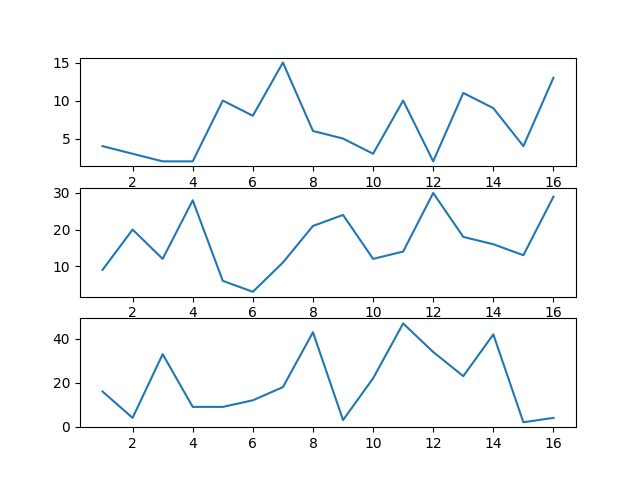Here a bit complex subplots

Here is the output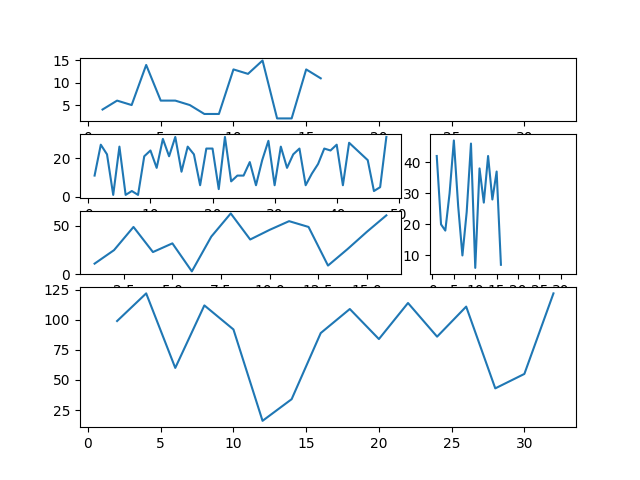Code for generating plot is as below

ax5 = plt.subplot2grid((5,3), (3, 0), colspan=3,rowspan=2,sharex=ax1)
• First bracket (5,3) indicates the number of rows and columns on the graph
• second bracket (3,0) indicates location by row, column. Since this is zero based indexing, (0,0) indicates first row and first column
• Plot can cover multiple rows and columns and can be specided by using colspan and rowspan
• Axis can be shared between two plots and its indicated by sharex

## Heatmap using Matplotlib and seaborn – Data Visualization using Python

A heatmap is a graphical representation of data where the individual values contained in a matrix are represented as colors. There are many different color schemes that can be used to illustrate the heatmap, with perceptual advantages and disadvantages for each. Rainbow colormaps are often used.

matplotlib as we as  seaborn can be used for creating heatmap. creating heatmap is bit difficult using matplotlib. Here is one example of using matplotlib for creating heatmap.

output is as belowHere is similar heatmap using searborn

Here is the output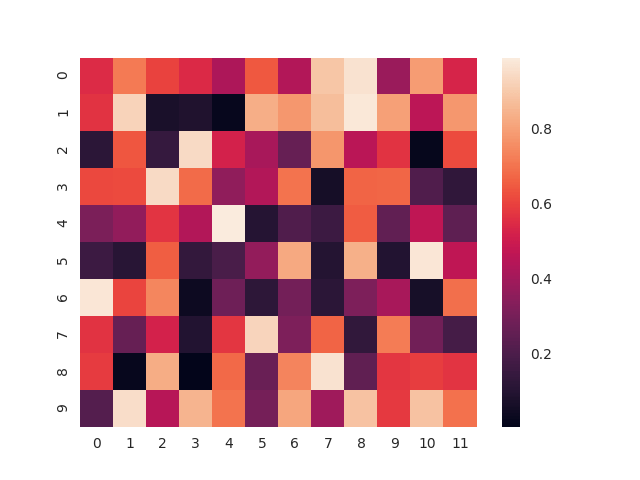As you can see, heatmap seems more refined and user friendly using seaborn than matplotlib. seaborn is not a replacement of matplotlib, its built on top of matplotlib only and these can be used together

Here is more complex heatmap

output is as belowUsing desired color code:

Parameter cmap is used to control the color code. You can use any of following parameters to get desired colors.

BuGn_r,Blues,BrBG,cubehelix,sns.cubehelix_palette(8),sns.light_palette(“green”),sns.light_palette(“navy”, reverse=False),YlGnBu

Also note that, you can define your own color scheme as well, please refer to official documentation for this here.

## How to add points to timeseries graph to show buy-sell signal – matplotlib

While doing time series analysis, you can show buy/sell signal on the ghraph itself.

Following is the script for doing the same.

While working on this code, I wasted quite a lot of time on below error.

Traceback (most recent call last):
File "timeseries_simple_with_pointer.py", line 35, in <module>
plt.scatter(df.loc[df['SMA20'] >1400.0 , 'Date'],df.loc[df['SMA20'] >1400.0, 'Close Price'], label='skitscat', color='red', s=25, marker="<")
File "/usr/local/lib/python3.6/site-packages/matplotlib/pyplot.py", line 3378, in scatter
edgecolors=edgecolors, data=data, **kwargs)
File "/usr/local/lib/python3.6/site-packages/matplotlib/__init__.py", line 1717, in inner
return func(ax, *args, **kwargs)
File "/usr/local/lib/python3.6/site-packages/matplotlib/axes/_axes.py", line 4023, in scatter
offsets = np.column_stack([x, y])
File "/usr/local/lib/python3.6/site-packages/numpy/lib/shape_base.py", line 369, in column_stack
return _nx.concatenate(arrays, 1)
TypeError: invalid type promotion

On further research I found that matplotlib scatter plot does not support pandas series and the scatter plot output needs to be converted into list so I had to use .values to make it work.

Output of my strategy using SMA 5 and SMAA12As you can see, in range bound market it creates a lot of whipsaw, however, it was able to capture a very good bull run.

I tried using larger duration SMA to see another strategy. Second time, I tried with SAM20 and SMA100 and here is the outputAs expected, longer duration SMA strategy provides lesser number of signals, lesser whipsaw’s.

This result does not mean you need to use higher duration SMA. I am planning to run this logic for approx 200 securities, will update this post with the feedabck later.

## How to plot simple and Candlestick chart using Python – pandas matplotlib

Pandas and Matplotlib can be used to plot various types of graphs. Simple timeseries plot and candlestick are basic graphs used by technical analyst for identifying the trend.

## Simple time Series Chart using Python – pandas matplotlib

Here is the simplest graph. It uses close price of HDFCBANK for last 24 months to plot normal graph

Here is the sample output## Candlestick chart using Python – pandas matplotlib

As per this link, module matplotlib.finance is deprecated in 2.0 and has been moved to a module called mpl_finance. This is still working when I have motplotlib version 2.1.2. This may stop working in any future releases, however, you can use mpl_finance module to use this feature. Please note that mpl_finance is no longer maintained.

Here is the output## Candlestick chart with SMA overlay using Python – pandas matplotlib

here is the output## Bar plots in Matplotlib – Data Visualization using Python

Matplotlib can be used to draw simple as well as complex bar plots. In this article we will see how to draw bar plots along with available options.

Here is an example of simple bar plot with available options.

Output of this script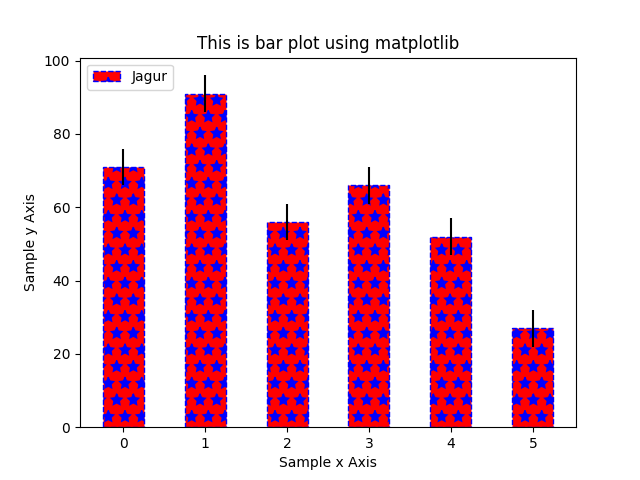Horizontal Bar plot

OutputStacked bar plot

Output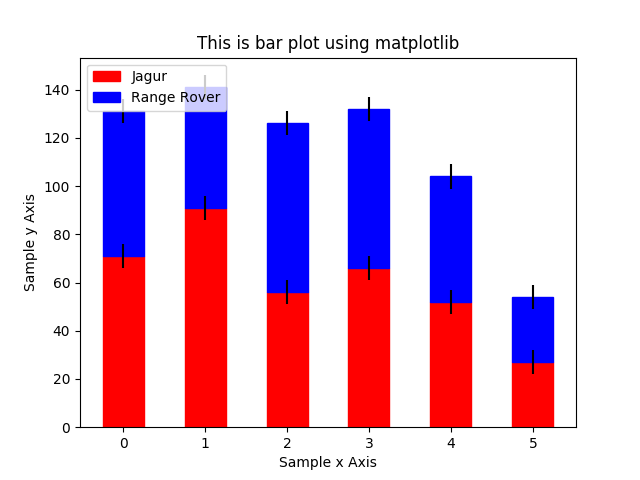bar plot with multiple data series

Output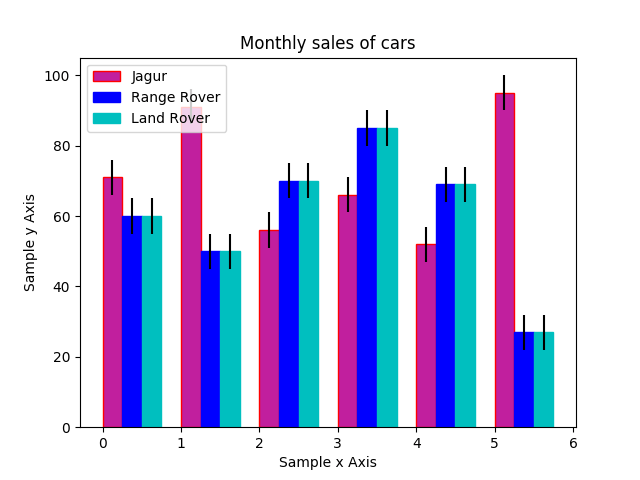## Histogram in Matplotlib – Data Visualization using Python

A histogram is an accurate representation of the distribution of numerical data. It is an estimate of the probability distribution of a continuous variable (quantitative variable)

A histogram is a plot that lets you discover, and show, the underlying frequency distribution (shape) of a set of continuous data. This allows the inspection of the data for its underlying distribution (e.g., normal distribution), outliers, skewness, etc.

To construct a histogram from a continuous variable you first need to split the data into intervals, called bins. In the example, age has been split into bins, with each bin representing a 10-year period or 5-year period.

### Histograms are based on area, not height of bars

In a histogram, it is the area of the bar that indicates the frequency of occurrences for each bin. This means that the height of the bar does not necessarily indicate how many occurrences of scores there were within each individual bin. It is the product of height multiplied by the width of the bin that indicates the frequency of occurrences within that bin

### What is the difference between a bar chart and a histogram?

The major difference is that a histogram is only used to plot the frequency of score occurrences in a continuous data set that has been divided into classes, called bins. Bar charts, on the other hand, can be used for a great deal of other types of variables including ordinal and nominal data sets.

Let us see how to plot histogram using python and maplotlib

Output is as below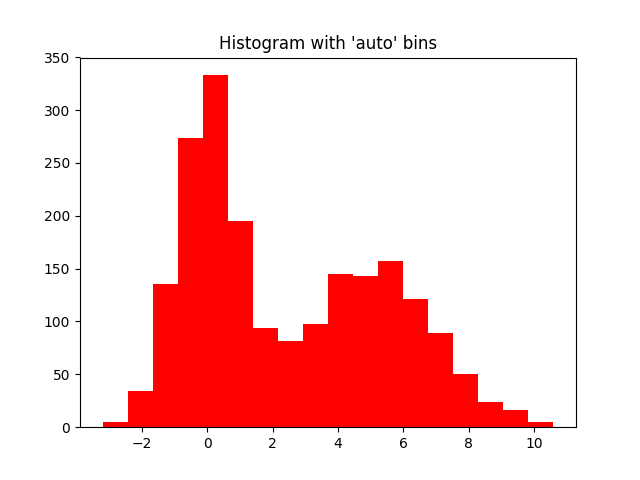Here is another example with additional details

Output is as belowYou can read further documentation here

If you want to change histogram to horizontal just change parameter orientation=horizontal

## How to use Subplots in matplotlib Data Visualization using Python

Many a times, I needed multiple plots in the same view as it helps in analyzing data in proper way. Also, if you want to present this data somewhere, it helps to plot two graphs together.

One alternative to subplots is using multiple axis and plotting 2 data points on the same graph but this might distort the view. Each has its own applications. In this article, we will look at how to plot  subplots.

Sytax is as below

subplot(nrows ncols index)

Here, nrows and ncols need not be same for all plots. Its only specific to current plot. Please refer to third example in this article

• Plotting three graphs using matplotlib

Output is as below• Plotting 4 graphs in 2*2 grid using matplotlibb

Output is as below• Plotting 3 graphs using matplotlib

Output is as belowIf you have noticed, in third example, subplot columns are different as compared to first one. This is because, we wanted to plot a single graph in second row.

Also note that we have used sharex=ax1, sharey=ax1 to maintain same scale on all columns. If scales are different, it does not gives us relative analysis.

## Scatter plots in Matplotlib – Data Visualization using Python

Scatter plots are one of the basic graphs used for data visualization. Scatter plot has following options, only first two are mandatory, rest all are optional.

plt.scatter(x, y, s=None, c=None, marker=None, cmap=None, norm=None, vmin=None, vmax=None, alpha=None, linewidths=None, verts=None, edgecolors=None, hold=None, data=None, **kwargs)

Here is simple program using scatter plot

Output of this program is as below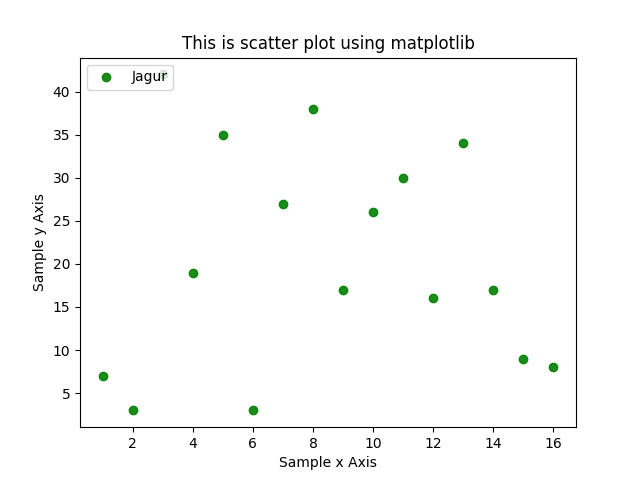Here is a bit complex scatter plot using matplotlib

Output of above program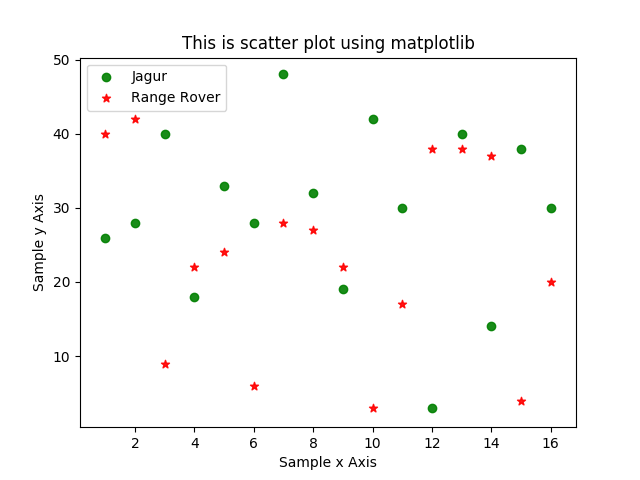You can read documentation about scatter plot here.

## What is pyplot and Line plots in Matplotlib – Data Visualization using Python

matplotlib.pyplot is a collection of command style functions that make matplotlib work like MATLAB. Each pyplot function makes some change to a figure: e.g., creates a figure, creates a plotting area in a figure, plots some lines in a plotting area, decorates the plot with labels, etc.

In this article, we will see how to use pyplot to draw line graph. Here is most basic plot

Here is the output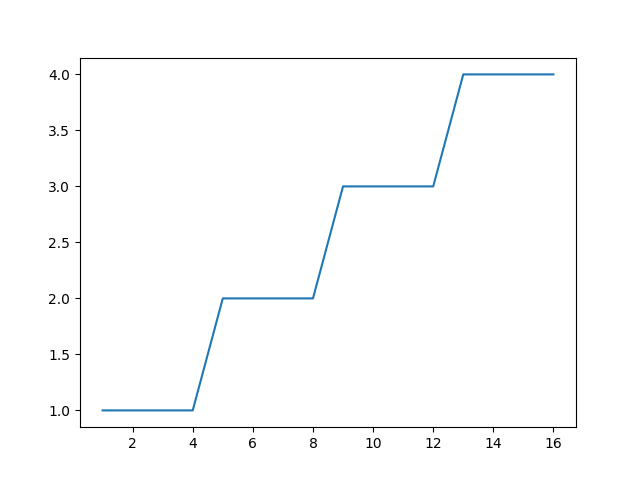You can add customization to line as below:

Line has various properties and these can be found here.

character description
'-' solid line style
'--' dashed line style
'-.' dash-dot line style
':' dotted line style
'.' point marker
',' pixel marker
'o' circle marker
'v' triangle_down marker
'^' triangle_up marker
'<' triangle_left marker
'>' triangle_right marker
'1' tri_down marker
'2' tri_up marker
'3' tri_left marker
'4' tri_right marker
's' square marker
'p' pentagon marker
'*' star marker
'h' hexagon1 marker
'H' hexagon2 marker
'+' plus marker
'x' x marker
'D' diamond marker
'd' thin_diamond marker
'|' vline marker
'_' hline marker

Color codes are as below

character color
‘b’ blue
‘g’ green
‘r’ red
‘c’ cyan
‘m’ magenta
‘y’ yellow
‘k’ black
‘w’ white